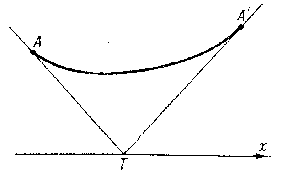# Lindelöf construction

A geometrical construction to find conjugate points in the problem of finding a minimal surface of revolution (see Fig.).Figure: l058950a

Lindelöf's construction remains suitable for any variational problem of the simplest type on the $(x,y)$-plane for which the general integral of the Euler equation can be represented in the form

$$\frac{y}{c_1}=f\left(\frac{x-c_2}{c_1}\right).$$

The tangents to the extremals at conjugate points $A$ and $A'$ intersect at some point $T$ on the $x$-axis, and the value of the variable integral along the arc $AA'$ is equal to its value on the polygonal line $ATA'$ (see ). An example is the catenoid with generating curve

$$\frac{y}{c_1}=\cosh\frac{x-c_2}{c_1}.$$

How to Cite This Entry:
Lindelöf construction. Encyclopedia of Mathematics. URL: http://encyclopediaofmath.org/index.php?title=Lindel%C3%B6f_construction&oldid=32354
This article was adapted from an original article by V.V. Okhrimenko (originator), which appeared in Encyclopedia of Mathematics - ISBN 1402006098. See original article